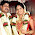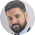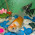# Time and Work problems with solutions

Time and Work is an important chapter for quantitative aptitude. Before solving questions below read my previous post on Time and Work concepts

I also made a video to teach basic concepts of this chapter. Questions in this chapter can be solved by two methods, first by fractions and secondly by finding efficiency in percentages. Both methods are equally good but by calculating efficiency in percentages, you can solve the questions quickly. In case you find any difficulty in any question, please leave a comment below.

1.
A can do a job 12 days, B is 60% more efficient than A. How many days B will take to complete the job alone ?
• A.
6 days
• C.
8 days
• E.
None of these
• B.
7 days
• D.
7.5 days
• Answer & Explanation
Explanation :
⇒ Efficiency of A = 1/12 = 8 ⅓ % per day
⇒ Efficiency of B per day =  8 ⅓ ×  1.6 = 40/3% per day
⇒ Number of days B needs to complete the job = 100 × 3/40 = 7.5 days

2.
Ram can finish a job in 18 days. Shaam can do the same job within 9 days. If they work togother what portoin of work they can complete in a day?
• A.
1/6
• C.
1/5
• B.
1/7
• D.
2/15
• Answer & Explanation

Explanation :
⇒ Ram can do 1/18 part of the job in one day
⇒ Shaam's efficiency is double so he can do 1/9 of the job per day
⇒ Together Ram and Shaam can do 1/18+1/9 = 1/6 of the job per day

3.
Adam can do a job in 15 days, Eve can do the same job in 20 days. If they work together for 4 days on this job. What fraction of job is incomplete ?
• A.
1/4
• C.
1/10
• B.
7/15
• D.
8/15
• Answer & Explanation
Explanation :
⇒ Adam can do 1/15 of the job per day
⇒ Eve can do 1/20 of the job per day
⇒ If they work together they can do 7/60 of the work together
⇒ Remaining job 1 - 7/60 = 32/60 = 8/15

4.
Ram takes 6 hours to type 32 pages. Shaam takes 5 hours to type 40 pages. In how many hours they will type 110 pages together ?
• A.
7 hours
• C.
8 hours
• B.
8 hours 15 min
• D.
8 hours 30 mins
• Answer & Explanation

Explanation :
⇒Ram can type 32/6 pages per hour
⇒ Shaam can type 8 pages per hour
⇒ They can type 32/6 + 8 = 40/3 pages per hour
⇒ So they will take 110/40 × 3 = 33/4 hours or 8 hours 15 minutes

5.
7 men take 12 days to complete a job. They worked for 5 days, after 5 days 2 men left the job. In how many days 5 men will complete the job ?
• A.
5 days
• C.
7 days
• B.
9 days
• D.
None of these
• Answer & Explanation

Explanation :
⇒7 men can do 1/12 of the job per day
⇒ So after 5 days they have completed 5/12th job. Still 7/12 part of the job is to be completed.
⇒ 5 men can do 1/12 × 5/7 = 5/84 of the job per day
⇒ So they will take 7/12 × 84 /5 = 9 ⅘ days to complete the job

6.
A can do ¼ of the job in 3 days. B can do ⅙ of the same job in 4 days. If they work together Rs 180 is to be paid to them in total, how much A should get ?
• A.
Rs 64
• C.
Rs 60
• B.
Rs 100
• D.
Rs 120
• Answer & Explanation

Explanation :
⇒A can do 1/12 part of the job per day
⇒ B can do 1/24 part of the job per day
⇒ A's efficiency is double than B so he must be paid double than B

7.
If A, B and C can do a job in 20, 30 and 60 days respectively. In how many days A can do the work if B and C help him on every third day ?
• A.
12 days
• C.
15 days
• B.
16 days
• D.
18 days
• Answer & Explanation

Explanation :
⇒Efficiency of A = 1/20 = 5% per day
⇒ Efficiency of B = 1/30 = 3.33% per day
⇒ Efficiency of C = 1/60 = 1.66% per day
⇒ In three days A can do 15% of the job himself and B and C do 5% of the job ( 1.66% + 3.33% )
⇒ In three days they can do 20% of the job, to do 100% of the job, they need 3 ×  5 = 15 days#### What's trending in BankExamsToday

Smart Prep Kit for Banking Exams by Ramandeep Singh - Download here

##### Join 40,000+ readers and get free notes in your email1.hi..here in question number 3
⇒ If they work together they can do 7/60 of the work together..but its said they work for 4 days together...this means 7/60*4=7/15
so remaning job will be =1-7/15 itself

1.You are doing it in a wrong way. Both of them can can do 4/15 + 2/20 = 5/15 of the work in 4 days.

2.chitravrenjith is right in her question

3.u r doing in wrong way 7/15 is done work so 8/15 is remaining work

4.Yes, something wrong here :)

Actually, it should be like this:
Fraction of work completed by both Adam &Eve working together in 1 day = 7/60
So, Fraction of work completed by both Adam &Eve working together in 4 days = 4* (7/60) = 28/60 = 7/15
So, 8/15 is the remaining work !!

2.In 1st question, y u took 1.6 in calculating efficiency of B..? not getting as how..!
60% means it will be 0.6.. right..?

1.Read carefully - "B is 60% more efficient" so his efficiency would be 160% of A.

2.A B
Efficiency 100 160
Days 160 100

So 12 x 100
------------------ = 7.5
160

3.I think now it is clear to you

3.Okay in first question it means efficiency is also calculated as a factor,
please see what i got from it, correct me if am wrong,
12 X 100 = (B's day) X 160 {100 & 160 r efficiencies respectively}
am i right?

1.Unable to understand your method

4.hi , can u pls tell me the ibps clerk cut off mark ......

1.tell me ua opn,sc, st or else so that wl tell u actual
.

5.sir can u explain last step of last question?

6.this is not a short cut method....

7.unable to understand 1st question can anyy one tell abt 1.6........

8.can any one explain abt 1.6.......... unable to underrstand

9.last step of last question sir. can u explain

10.can u please explain the last step in ques 5

11.i couldn't unerstan the last two lines of explanation for the last that is the 7 th problem ....can u plzzzz explain it once again?

12.pls answer this question in efficient shortcut method.
A can do a certain job in 12 days.B is 60% more efficient than A.How many days does B alone take to do the same job?

13.For the last question
A's one days eff. is 5% so A's 3 days is 15%
B + C's one days eff. is 5%.
so A+B+C's 3days eff. is 20%.
now
3days ------ 20%
? ------- 100%
300/20 = 15

Thanks for commenting. Follow us on Telegram. Search BankExamsToday